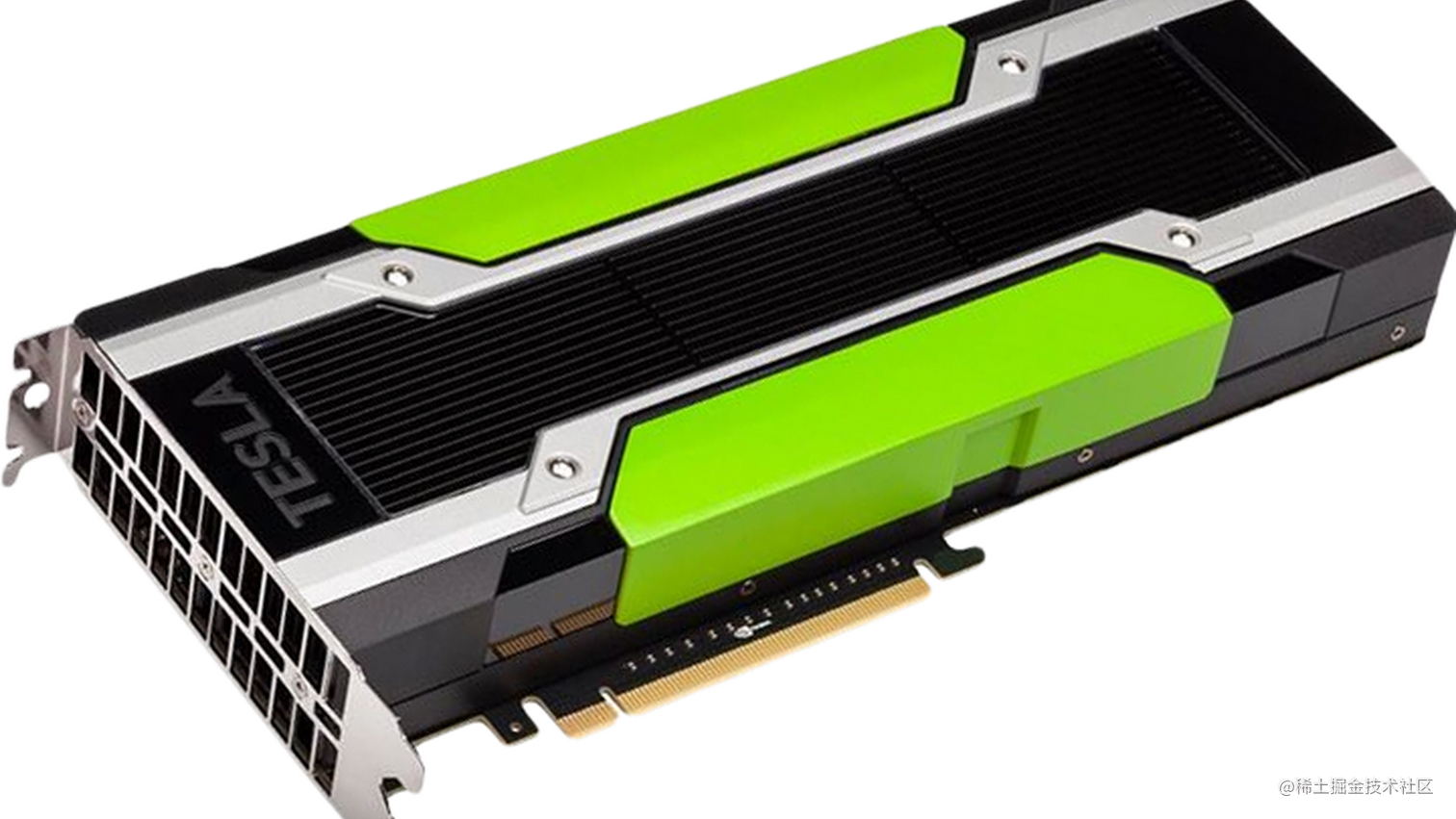# [译] Google Colab 免费 GPU 使用教程# Google Colab 免费 GPU 使用教程

### 感谢 KDnuggets！

• 提高你的 Python 语言的编码技能。
• 使用 KerasTensorFlowPyTorchOpenCV 等流行库开发深度学习应用程序。

Colab 与其它免费的云服务最重要的区别在于：Colab 提供完全免费的 GPU。

### 在 Google Colab 中运行或导入 .py 文件

``````from google.colab import drive
drive.mount('/content/drive/')

``````!ls "/content/drive/My Drive/"

``````!pip install -q keras

mnist_cnn.py 文件内容

MNIST 数据集上运行下面的代码来训练一个简单的卷积网络（convnet）。

``````!python3 "/content/drive/My Drive/app/mnist_cnn.py"

### 下载 Titanic 数据集（.csv 文件）并显示文件的前 5 行内容

!wget raw.githubusercontent.com/vincentarel… -P "/content/drive/My Drive/app"

``````import pandas as pd

### 克隆 GitHub 仓库到 Google Colab

#### 2. 使用 Git 克隆

!git clone github.com/wxs/keras-m…

### 一些有用的提示

#### 1. 如何安装第三方库？

Keras

``````!pip install -q keras
import keras

PyTorch

``````from os import path
from wheel.pep425tags import get_abbr_impl, get_impl_ver, get_abi_tag
platform = '{}{}-{}'.format(get_abbr_impl(), get_impl_ver(), get_abi_tag())
accelerator = 'cu80' if path.exists('/opt/bin/nvidia-smi') else 'cpu'

import torch

`!pip3 install torch torchvision`

MxNet

``````!apt install libnvrtc8.0
!pip install mxnet-cu80
import mxnet as mx

OpenCV

``````!apt-get -qq install -y libsm6 libxext6 && pip install -q -U opencv-python
import cv2

XGBoost

``````!pip install -q xgboost==0.4a30
import xgboost

GraphViz

``````!apt-get -qq install -y graphviz && pip install -q pydot
import pydot

7zip 阅读器

``````!apt-get -qq install -y libarchive-dev && pip install -q -U libarchive
import libarchive

`!pip install` 或者 `!apt-get install` 安装其它库。

#### 2. GPU 是否正常工作？

``````import tensorflow as tf
tf.test.gpu_device_name()

#### 3. 我使用的是哪一个 GPU？

``````from tensorflow.python.client import device_lib
device_lib.list_local_devices()

#### 4. 输出 RAM 信息？

``````!cat /proc/meminfo

#### 5. 输出 CPU 信息？

``````!cat /proc/cpuinfo

#### 6. 改变工作文件夹

``````!ls

``````import os
os.chdir("drive/app")
# 译者注：挂载网盘目录后，前面没有切换过目录，这里应该输入
# os.chdir("drive/My Drive/app")

``````!ls

#### 7. “`No backend with GPU available`” 错误解决方案

Failed to assign a backend No backend with GPU available. Would you like to use a runtime with no accelerator? #指定后端失败。没有可用的 GPU 后端。需要使用没有加速器的运行时吗？

#### 9. “apt-key output should not be parsed (stdout is not a terminal)” 警告

``````Warning: apt-key output should not be parsed (stdout is not a terminal) #警告：apt-key 输出无法被解析（当前 stdout 不是终端）

``````!mkdir -p drive

#### 10. 如何在 Google Colab 中使用 Tensorboard？

github.com/mixuala/col…

``````!kill -9 -1

#### 14. 如何将大文件从 Colab 发送到 Google Drive？

``````# 需要发送哪个文件？
file_name = "REPO.tar"

auth.authenticate_user()
drive_service = build('drive', 'v3')

def save_file_to_drive(name, path):
file_metadata = {'name': name, 'mimeType': 'application/octet-stream'}

return created

save_file_to_drive(file_name, file_name)

#### 15. 如何在 Google Colab 中运行 Tensorboard？

``````# 你可以更改目录名
LOG_DIR = 'tb_logs'

!wget https://bin.equinox.io/c/4VmDzA7iaHb/ngrok-stable-linux-amd64.zip
!unzip ngrok-stable-linux-amd64.zip

import os
if not os.path.exists(LOG_DIR):
os.makedirs(LOG_DIR)

get_ipython().system_raw(
'tensorboard --logdir {} --host 0.0.0.0 --port 6006 &'
.format(LOG_DIR))

get_ipython().system_raw('./ngrok http 6006 &')

!curl -s http://localhost:4040/api/tunnels | python3 -c \

``````from __future__ import print_function
import keras
from keras.datasets import mnist
from keras.models import Sequential
from keras.layers import Dense, Dropout, Flatten
from keras.layers import Conv2D, MaxPooling2D
from keras import backend as K
from keras.callbacks import TensorBoard

batch_size = 128
num_classes = 10
epochs = 12

# 输入图像维度
img_rows, img_cols = 28, 28

# the data, shuffled and split between train and test sets
(x_train, y_train), (x_test, y_test) = mnist.load_data()

if K.image_data_format() == 'channels_first':
x_train = x_train.reshape(x_train.shape, 1, img_rows, img_cols)
x_test = x_test.reshape(x_test.shape, 1, img_rows, img_cols)
input_shape = (1, img_rows, img_cols)
else:
x_train = x_train.reshape(x_train.shape, img_rows, img_cols, 1)
x_test = x_test.reshape(x_test.shape, img_rows, img_cols, 1)
input_shape = (img_rows, img_cols, 1)

x_train = x_train.astype('float32')
x_test = x_test.astype('float32')
x_train /= 255
x_test /= 255
print('x_train shape:', x_train.shape)
print(x_train.shape, 'train samples')
print(x_test.shape, 'test samples')

# 将类别向量转换成二分类矩阵
y_train = keras.utils.to_categorical(y_train, num_classes)
y_test = keras.utils.to_categorical(y_test, num_classes)

model = Sequential()
activation='relu',
input_shape=input_shape))

model.compile(loss=keras.losses.categorical_crossentropy,
metrics=['accuracy'])

tbCallBack = TensorBoard(log_dir=LOG_DIR,
histogram_freq=1,
write_graph=True,
batch_size=batch_size,
write_images=True)

model.fit(x_train, y_train,
batch_size=batch_size,
epochs=epochs,
verbose=1,
validation_data=(x_test, y_test),
callbacks=[tbCallBack])
score = model.evaluate(x_test, y_test, verbose=0)
print('Test loss:', score)
print('Test accuracy:', score)

Tensorboard :)